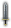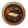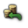贸易策略

控制贸易节点

节点贸易力量占比

• 派遣轻帆船进行贸易护航任务，并设法击沉其他国家的轻帆船。
• 铺设贸易加成建筑，由于某些建筑提供百分比加成，请于有诸如天然良港之类的省份优先建设。
• 征服或殖民节点内的省份。
• 对节点中的其他国家实施禁运。

理想的贸易节点

• 拥有较高的贸易价值，可以是来自于省份生产，也可以是来自于上游节点的贸易引导。
• 存在的竞争较少，这样在掌控节点上所消耗的资源也更少。
• 与已经掌握的节点相连，这样可以最大化贸易引导的收益。
• 终点节点，不会因为引导而使贸易值流失。
• 贸易本埠的下游节点。
• 贸易本埠所在节点，提升贸易力最为经济。

• 亚丁湾节点是贸易值由中国与印度由海路流向欧洲的咽喉要道. 它决定了一笔庞大的贸易额究竟是流向亚历山大港和地中海，或是霍尔木兹与中东，或是绕非洲一周后前往欧洲西部。（与之相同的是印度河节点，虽然与亚丁湾节点相连，但从这里流出的都是抄近路前往中东的贸易额，很少会有流向欧洲的贸易额）。
• 象牙海岸 节点决定了来自亚洲和非洲的贸易额是流向美洲，突尼斯，或其他众多的欧洲节点（塞维利亚，波尔多或者英吉利海峡）。
• 来自美洲的贸易额大多会集中在东海岸，而后前往加勒比海节点. 这个节点决定了这些贸易额是直接流入塞维利亚，波尔多（同时接受来自加勒比海和圣劳伦斯湾的贸易额），英吉利海峡（经过切斯比克湾），或是北海与丹麦（经过圣劳伦斯湾）。
• 尽管东亚没有终点节点，但几乎所有的贸易额都会通过这两个节点，北方的北京和南方的马六甲，之后再经过某国的贸易本埠.如果军力足够，可以考虑推进至桑吉巴尔或者印度洋节点来扩大利润，甚至可以考虑染指亚丁湾
• 中亚诸国可以依靠内陆节点的加成来围绕丝绸之路渔利，同时接受中国中部和亚洲草原部落的贸易值并输向波斯地区的撒马尔罕是一个非常有价值的节点。

贸易禁运

• 如果一国对禁运另一国有很强欲望，并愿意受关系惩罚，那么大概不介意宣布被禁运方为宿敌。
• 禁运非宿敌的贸易收益至少与受到的 -50% 贸易效率惩罚持平，如果是这样，需要你的禁运对象体量够大，实力够强——那为什么不宣做宿敌？
• 禁运宿敌会提升力量投射，而禁运非宿敌不会。

• 本国节点贸易力量比率虽大但不至垄断——50%到80%之间比较合适，一国贸易竞争力占比为65.4%时，禁运所有节点中其他国家，贸易竞争力占比会提升8.3%——提升至73.7%，这也是禁运能造成的最大即时贸易力占比提升。
• 由于禁运非宿敌会遭受贸易效率惩罚，所以谨慎选择你想要同时禁运的对象。
• 目标国家掌握了节点中几乎剩下的份额。

• 在本国收集贸易值的节点，所有节点中拥有贸易力占比的其他国家都是你的竞争对手。
• 在本国引导贸易的节点，其他正在节点中进行贸易引导的国家是你的竞争对手。
• 在一些重要的节点，禁运甚至会对全球贸易的形势产生重要影响，谨慎选择你的禁运对象，因为一些国家会在某些节点共享利益，而在另一些节点中互相竞争。

指派商人团

在本埠收集贸易值

DLC国富论中，贸易本埠（或称主要贸易节点）会自动收集贸易值，无论该节点是否被指派了商人团. 在贸易本埠派遣商人团收集贸易额会给予 +10%贸易收入修正，但仅作用于这一本埠节点所产生的贸易收入.除非本国拥有贸易额的节点少于商人团数量，在贸易本埠额外派遣商人收集贸易额在大多数情况下是不值得的

转移贸易额

• 目标节点目前的贸易额流向。如果一个节点的贸易额已经流向了对本国有利的方向，或者节点贸易额的流向是单一的，就没有必要再派商人团转移贸易额。

• 目标节点对本国的价值。一国向节点派遣轻帆船保护贸易的前提是在目标节点拥有贸易力量占比，或者是在目标节点部署了商人团，你可以先向节点派出商人团，然后再派出轻帆船，从而影响一个本国之前并没有贸易力的节点。

• 目标节点的位置。在一条被本国控制的贸易链条的末端进行引导可以最大化收益。

• 节点中是否有其他商人团在引导贸易.节点中多于一个商人团的情况下，加成会随商人团数量的增加而减少。

引流，还是收集？

• 如果一国在下游没有部署商人团，或者下游不是本国的贸易本埠，那么应该在这个节点收集贸易额
• 如果一国控制了一条通向本埠或者其他正在收集贸易值的节点，那么应该在沿途的节点引导贸易，赚取额外净收益。
• 如果商人团数量和节点控制力允许，让贸易值通过尽可能多的节点有利于增加额外净收益，从而增加最后在收集节点得到的贸易额。
• 尽可能不要向上游转移贸易额，-80% 的贸易值惩罚使这个行动在一般情况下毫无意义。

• 热那亚
• 英吉利海峡
• 威尼斯

边际收益

$\frac{d \left( \text{ 拥 有 的 贸 易 力 量 占 比 } \right)}{d \left( \text{ 拥 有 的 贸 易 力 量 } \right)} = \frac{1 - \text{ 目 前 拥 有 的 贸 易 力 量 占 比 } }{\text{ 节 点 贸 易 力 量 总 额 }}$

收集贸易额的情况

${ \frac{d \left( \text{ 本 国 贸 易 收 入 } \right)}{d \left( \text{ 本 国 节 点 贸 易 力 量 } \right)} = (100\% + \text{ 贸 易 收 入 修 正 }) \cdot \text{ 节 点 贸 易 值 总 额 } \cdot \frac{1 - \text{ 本 国 节 点 贸 易 力 量 占 比 } }{\text{ 节 点 贸 易 力 量 总 额 }} }$

d(R)/d(P) = (100% + r) * V * (1 - P) / P
d(R)/d(P) = (100% + 0) * 10 * (1 - .25) / 150
d(R)/d(P) = .05

d(R)/d(P) = (100% + r) * V * (1 - P) / P
d(R)/d(P) = (100% + 0) * 10 * (1 - (37.5+100)/250) / (150+100)
d(R)/d(P) = (100% + 0) * 10 * (1 - .55) / 250
d(R)/d(P) = .018

流出的贸易额

$\frac{d \left( \text{ 流 出 贸 易 额 } \right)}{d \left( \text{ 用 于 引 流 的 贸 易 力 量 占 比 } \right)} = \text{ 节 点 总 贸 易 值 } \cdot \frac{1 - \text{ 用 于 引 流 的 贸 易 力 量 } }{\text{ 节 点 总 贸 易 力 量 }}$

${ \frac{d \left( \text{ 向 某 方 向 被 引 导 的 总 贸 易 值 } \right)}{d \left( \text{ 用 于 向 某 方 向 引 导 的 贸 易 力 量 } \right)} = (1 + \text{ 复 数 商 人 加 成 }) \cdot \text{ 节 点 总 贸 易 值 } \cdot \text{ 用 于 引 流 的 贸 易 力 量 占 比 } \cdot \frac{1 - \text{ 用 于 向 某 方 向 引 导 的 贸 易 力 量 占 比 } }{\text{ 用 于 向 某 方 向 引 导 的 贸 易 力 量 总 和 }} }$

另见

 概括 建筑 • 殖民 • 贸易 • 产品 • 贸易节点 • 贸易公司 • 殖民领 • 发展度 • 税收 • 生产 • 过度扩张 • 厌战度